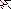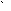# MINLPLib

### A Library of Mixed-Integer and Continuous Nonlinear Programming Instances

#### Instance gkocis

 Formatsⓘ ams gms mod nl osil py Primal Bounds (infeas ≤ 1e-08)ⓘ -1.92309874 p1 ( gdx sol ) (infeas: 2e-14) Other points (infeas > 1e-08)ⓘ Dual Boundsⓘ -1.92309874 (ANTIGONE)-1.92309874 (BARON)-1.92309874 (COUENNE)-1.92309874 (LINDO)-1.92309874 (SCIP)-1.92309925 (SHOT) Referencesⓘ Kocis, Gary R and Grossmann, I E, Relaxation Strategy for the Structural Optimization of Process Flow Sheets, Industrial and Engineering Chemistry Research, 26:9, 1987, 1869-1880. Sourceⓘ MINOPT Model Library model kocis87-2.dat Added to libraryⓘ 01 May 2001 Problem typeⓘ MBNLP #Variablesⓘ 11 #Binary Variablesⓘ 3 #Integer Variablesⓘ 0 #Nonlinear Variablesⓘ 2 #Nonlinear Binary Variablesⓘ 0 #Nonlinear Integer Variablesⓘ 0 Objective Senseⓘ min Objective typeⓘ linear Objective curvatureⓘ linear #Nonzeros in Objectiveⓘ 8 #Nonlinear Nonzeros in Objectiveⓘ 0 #Constraintsⓘ 8 #Linear Constraintsⓘ 6 #Quadratic Constraintsⓘ 0 #Polynomial Constraintsⓘ 0 #Signomial Constraintsⓘ 0 #General Nonlinear Constraintsⓘ 2 Operands in Gen. Nonlin. Functionsⓘ log Constraints curvatureⓘ indefinite #Nonzeros in Jacobianⓘ 19 #Nonlinear Nonzeros in Jacobianⓘ 2 #Nonzeros in (Upper-Left) Hessian of Lagrangianⓘ 2 #Nonzeros in Diagonal of Hessian of Lagrangianⓘ 2 #Blocks in Hessian of Lagrangianⓘ 2 Minimal blocksize in Hessian of Lagrangianⓘ 1 Maximal blocksize in Hessian of Lagrangianⓘ 1 Average blocksize in Hessian of Lagrangianⓘ 1.0 #Semicontinuitiesⓘ 0 #Nonlinear Semicontinuitiesⓘ 0 #SOS type 1ⓘ 0 #SOS type 2ⓘ 0 Minimal coefficientⓘ 9.0000e-01 Maximal coefficientⓘ 1.1000e+01 Infeasibility of initial pointⓘ 0 Sparsity JacobianⓘSparsity Hessian of Lagrangianⓘ```\$offlisting
*
*  Equation counts
*      Total        E        G        L        N        X        C        B
*          9        6        0        3        0        0        0        0
*
*  Variable counts
*                   x        b        i      s1s      s2s       sc       si
*      Total     cont   binary  integer     sos1     sos2    scont     sint
*         12        9        3        0        0        0        0        0
*  FX      0
*
*  Nonzero counts
*      Total    const       NL      DLL
*         28       26        2        0
*
*  Solve m using MINLP minimizing objvar;

Variables  x1,x2,x3,x4,x5,x6,x7,x8,b9,b10,b11,objvar;

Positive Variables  x1,x2,x3,x4,x5,x6,x7,x8;

Binary Variables  b9,b10,b11;

Equations  e1,e2,e3,e4,e5,e6,e7,e8,e9;

e1..  - 1.8*x1 - 7*x5 - x6 - 1.2*x7 + 11*x8 - 3.5*b9 - b10 - 1.5*b11 + objvar
=E= 0;

e2.. -log(1 + x2) + x6 =E= 0;

e3.. -1.2*log(1 + x3) + x7 =E= 0;

e4..  - 0.9*x4 + x8 =E= 0;

e5..  - x4 + x5 + x6 + x7 =E= 0;

e6..    x1 - x2 - x3 =E= 0;

e7..    x4 - 5*b9 =L= 0;

e8..    x2 - 5*b10 =L= 0;

e9..    x3 - 5*b11 =L= 0;

* set non-default bounds
x6.up = 5;
x8.up = 1;

Model m / all /;

m.limrow=0; m.limcol=0;
m.tolproj=0.0;

\$if NOT '%gams.u1%' == '' \$include '%gams.u1%'

\$if not set MINLP \$set MINLP MINLP
Solve m using %MINLP% minimizing objvar;

```

Last updated: 2023-08-16 Git hash: 2519540e